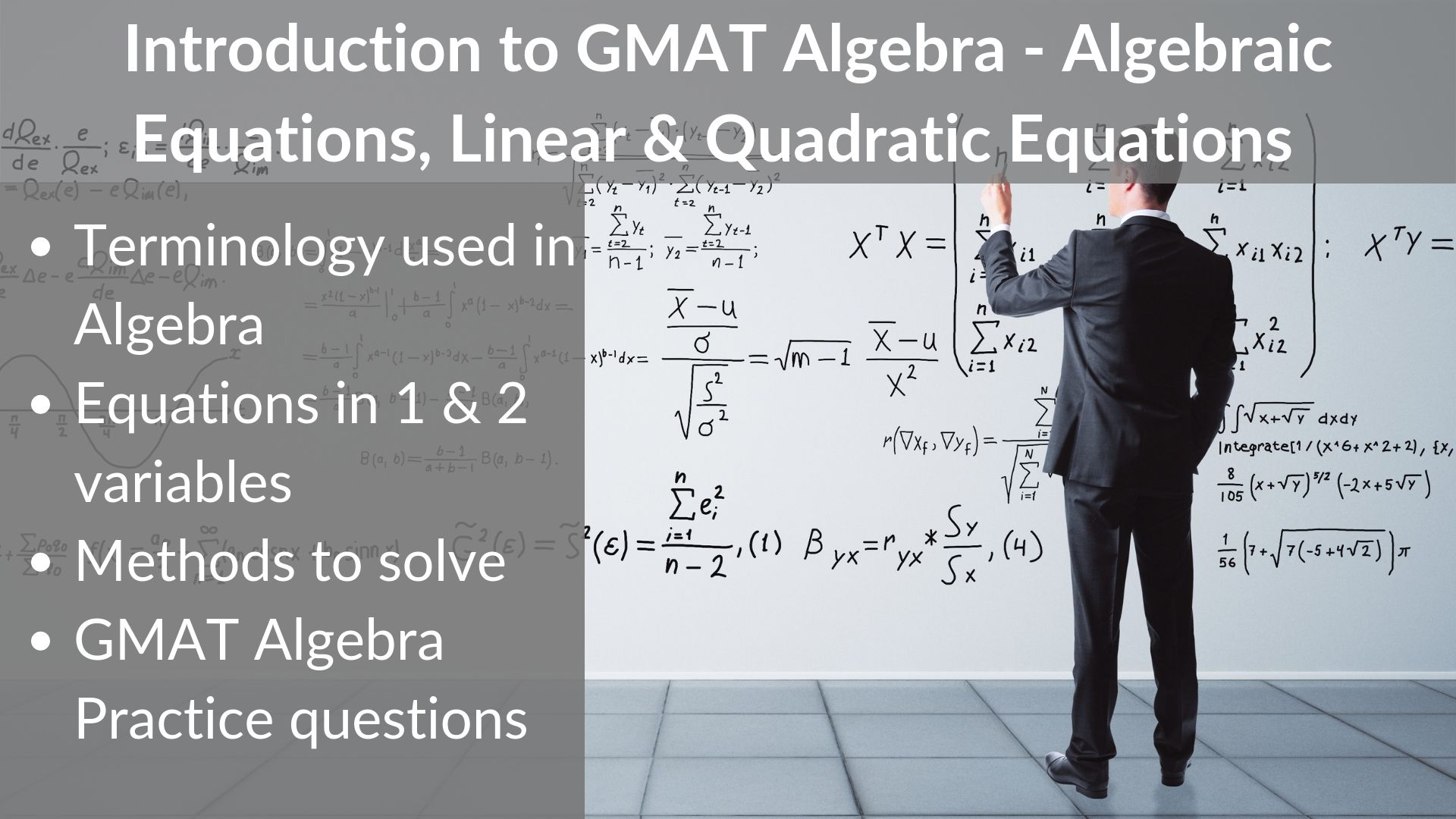# The total price of 5 pounds of regular coffee and 3 pounds of decaffeinated coffee was \$21.50… – OG 2020 Question #315 with Solution

A 2 minute read

OG 2020: Question No. 315 The total price of 5 pounds of regular coffee and 3 pounds of decaffeinated coffee was \$21.50. What was the price of the 5 pounds of regular coffee? If the price of the 5 pounds of regular coffee had been reduced 10 percent and the price of the 3 pounds

Read More# If a and b are integers, is a5 < 4b? - OG 2020 Question #316 with Solution

A < 1 minute read

OG 2020: Question No. 316 If a and b are integers, is a5 < 4b? a3 = –27 b2 = 16 Source OG 2020 Type Data Sufficiency Topic Algebra Sub-Topic Exponents Difficulty Medium Solution Steps 1 & 2: Understand Question and Draw Inferences In this question, we are given The numbers a and b are

Read More# If r and s are positive numbers and θ is one of the operations, +, −, ×, or ÷, which operation is θ? – OG 2020 Question #311 with Solution

A 2 minute read

OG 2020: Question No. 311 If r and s are positive numbers and θ is one of the operations, +, −, ×, or ÷, which operation is θ? If r = s, then r θ s = 0. If r ≠ s, then r θ s ≠ s θ r. Source OG 2020 Type Data

Read More# If x, y, and k are positive and x is less than y, then (x + k) / (y + k)  is – OGQR 2020 Question #80 with Solution

A < 1 minute read

OGQR 2020: Question No. 80 If x, y, and k are positive and x is less than y, then (x + k) / (y + k)  is Source OGQR 2020 Type Problem Solving Topic Algebra Sub-Topic Ratio / Inequality Difficulty Medium Solution Given In this question, we are given The numbers x, y, and k

Read More# GMAT Algebra and Algebraic Expressions | Linear & Quadratic Equations

A 9 minute read

The word Algebra was derived from the Arabic word “al-jabr” meaning “reunion of broken parts”.  In algebra, arithmetic computations are carried out with letters standing for numbers. Many students find algebra difficult and through this article, we aim to demystify GMAT Algebra and how you can solve GMAT Algebra questions with ease.   Simple Algebraic

Read More# Consider the following set of inequalities: p > q, s > r, q > t, s > p, and r > q. Between which two quantities is no relationship established? – OGQR 2020 Question #81 with Solution

A < 1 minute read

OGQR 2020: Question No. 81 Consider the following set of inequalities: p > q, s > r, q > t, s > p, and r > q. Between which two quantities is no relationship established? Source OGQR 2020 Type Problem Solving Topic Algebra Sub-Topic Inequality Difficulty Medium Solution Given In this question, we are given

Read More# In any sequence of n nonzero numbers, a pair of consecutive terms with opposite signs represents a sign change. For example, the sequence –2, 3, –4, 5 has three sign changes. Does the sequence of nonzero numbers s1, s2, s3, . . ., sn have an even number of sign changes? – OG 2020 Question #312 with Solution

A 2 minute read

OG 2020: Question No. 312 In any sequence of n nonzero numbers, a pair of consecutive terms with opposite signs represents a sign change. For example, the sequence –2, 3, –4, 5 has three sign changes. Does the sequence of nonzero numbers s1, s2, s3, . . ., sn have an even number of sign

Read More# If half the result obtained when 2 is subtracted from 5x is equal to the sum of 10 and 3x, what is the value of x? – OGQR 2020 Question #77 with Solution

A < 1 minute read

[two_third] OGQR 2020: Question No. 77 If half the result obtained when 2 is subtracted from 5x is equal to the sum of 10 and 3x, what is the value of x? [/two_third] [one_third_last] Source OGQR 2020 Type Problem Solving Topic Algebra Sub-Topic Linear Equation Difficulty Easy-Medium [/one_third_last] Solution Given In this question, we are

Read More# How old is Jane? – OGQR 2020 Question #208 with Solution

A < 1 minute read

[two_third] OGQR 2020: Question No. 208 How old is Jane? Ten years ago, she was one-third as old as she is now. In 15 years, she will be twice as old as she is now. [/two_third] [one_third_last] Source OGQR 2020 Type Data Sufficiency Topic Algebra Sub-Topic Linear Equations Difficulty Easy [/one_third_last] Solution Steps 1 &

Read More# Last year Publisher X published 1,100 books, consisting of first editions, revised editions, and reprints. How many first editions did Publisher X publish last year? – OGQR 2020 Question #207 with Solution

A 2 minute read

[two_third] OGQR 2020: Question No. 207 Last year Publisher X published 1,100 books, consisting of first editions, revised editions, and reprints. How many first editions did Publisher X publish last year? The number of first editions published was 50 more than twice the number of reprints published. The number of revised editions published was half the number of

Read More